# Data Structures & Algorithms 101: Bit Manipulation in JavaScript Part 1

Welcome back fellow software engineers! If you are new to my blog, it’s great to have you here and I hope you’re able to gain a little something from it. But, this week, I’m coming back to Data Structures and Algorithms 101 so I can talk about Bit Manipulation! Bit manipulation is used in a multitude of problems and is another extremely important algorithm that you need in your tool box to conquer those code challenges! There is a lot to Bit Manipulation so I am splitting this topic into two blogs! This week I’m going to give you a run down of the basics of bits and then go over some of the basic bitwise operations in JavaScript. I will provide some examples, which you can follow along in your browser console.

# What Are Bits and Why Are They Important

A bit or “binary digit”, is the most basic representation of data for computers. There are only two possible values used to represent a binary digit, 1 and 0. A combination of 1’s and 0’s are used to represent numbers with bits, such as the number 26. We can represent 26 by dividing the number by 2 and then using the remainder to determine whether to use a 0 or 1. Then we display our number in reverse order from what we calculated to provide the binary representation of the number. Let’s break it down in the example below.

• 13/2 = 6, remainder: 1
• 6/2 = 3, remainder: 0
• 3/2 = 1, remainder: 1
• 1/2 = 0, remainder: 1

# How Do We Represent Binaries in JavaScript

As part of the ES6 rollout, representing numbers in different bases in JavaScript requires us to start the number literal with 0 followed by a letter. For binaries, we start a representation with 0b. For example, if we want to represent 11010 (26) as a binary number, you would write:

## toString()

You might have seen this method before when attempting to turn JavaScript objects into a string. But, I will show you how you can use it to find the binary representation of a number or any other based representation(up to 36) of a decimal. Let’s use the number 26 like we did above and check to see if we get the same binary representation that we calculated. Now to convert, we write the syntax like this: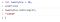toString() method to turn a decimal number into a binary number

## parseInt()

Now, let’s see how we can transform numbers using the parseInt() method! This is more often used to convert numbers of different bases to Number primitives. The syntax for the parseInt() method is: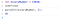Using parsInt() method to convert 11010 binary number to a decimal

# Bitwise Operations and How To Manipulate Bits

Now that we know what bits are and how to transform any integer to a binary number, let’s get into some different operations that will help us manipulate bits and have them work for us. I will be using the operations in examples and I encourage you to follow along!

## Slight But Important Detour

Before we get started with bitwise operations, there is an important note about binary numbers that I wanted to provide to you. I want to discuss how we write a negative number in binary. When establishing whether a binary number is positive or negative, we use what’s called a signed number. Signed binary numbers use the leftmost digit to determine whether the the number is positive or negative. We can also refer to the leftmost digit as the MSB or “most significant bit”. If the binary number is negative the MSB will be 1, and if it is positive the MSB will be 0. Now that you know how to represent negative and positive binary numbers let’s go through some different bitwise operations that will help you manipulate some bits!

## Bitwise Operations

First, it’s important to know that in JavaScript when bitwise operations are performed the numbers are converted from 64-bit numbers to 32-bit signed integers. So when I told you earlier that JavaScript represents numbers using 64-bits, once a bitwise operation is used to manipulate that number, it is converted into a 32-bit signed number. It’s important to keep this in mind in case the representations of numbers you use at first have a different number of bits after you use the bitwise operations. JavaScript does this to guarantee that binary numbers that you operate with will always have the same number of bits and help you identify positive and negative integers. Now, let’s start with the & (AND) operator!

## & (AND) Operator

The bitwise AND operator (&), when applied to 2 binary numbers, will return 1 whenever the corresponding bits of both are 1. Let’s look at our example below to get a better understanding of what’s happening!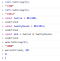Using the & bitwise operator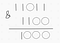How we got the binary number for 8 from twelve & twentySeven

## | (OR) Operator

The OR operator (|) is a bitwise operator that will return 1 whenever one of the corresponding bits of the operand is 1. Let’s use the same example as above, but use the OR operator to see how it works.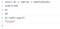OR bitwise Operator returning 31 or 11111.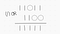Using the OR operator to return the answer of 31

## ^(XOR) Operator

The XOR operator, also known as the exclusive OR, is an operator that will return 1 whenever the two corresponding bits are different from each other. This means that any combination of zeros and ones will result in 1. Let’s take our example above and use the XOR operator on twelve and twentySeven.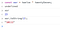XOR operator being used on twelve and twentySeven to get 23 or 10111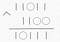Drawing board proof of how the XOR operator works on binary numbers

## ~(NOT) Operator

The last operator I want to go over in this blog is the NOT operator. It is a pretty simple operator because all it does is invert the operand. This means when the digit is 1 it will return a 0 and vice versa. Let’s look at the NOT operator in action with our example.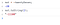Using the NOT Operator on twentySeven

# Conclusion

In this week’s blog we’ve learned what bits are and why they are important. We also began reviewing some of the basic bitwise operations for bit manipulation in JavaScript. I hope this blog was informational and helpful for you as you continue learning about data structures and algorithms. Part 2 will come out next week and discuss some more advanced operations that will review shifting and adding and subtracting binary numbers. As always, I challenge you to continue researching and practicing bit manipulation and come back next week for part 2!